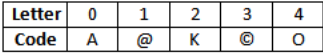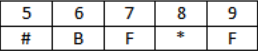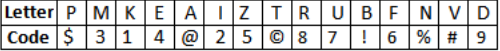Courses

# Alphanumeric Series MCQ - 2

## 30 Questions MCQ Test General Intelligence and Reasoning for SSC CHSL | Alphanumeric Series MCQ - 2

Description
This mock test of Alphanumeric Series MCQ - 2 for LR helps you for every LR entrance exam. This contains 30 Multiple Choice Questions for LR Alphanumeric Series MCQ - 2 (mcq) to study with solutions a complete question bank. The solved questions answers in this Alphanumeric Series MCQ - 2 quiz give you a good mix of easy questions and tough questions. LR students definitely take this Alphanumeric Series MCQ - 2 exercise for a better result in the exam. You can find other Alphanumeric Series MCQ - 2 extra questions, long questions & short questions for LR on EduRev as well by searching above.
QUESTION: 1

### Directions (1-5): In each question below is given a group of digits followed by four combinations of digits/symbols given in (A), (B), (C) and (D). You have to find out which of the combinations correctly represents the group of digits based on the rules and mark the answer accordingly. If none of the combinations correctly represents the group of letters, mark (E) as your answer. (i) If the first digit is odd and last is even, then interchange the codes for both digits (ii) If there is a digit which is getting repeated in the given number, then code that digit as the code of 5 (iii) If the third digit is followed by an even number and is preceded by an odd number, then skip its code. (iv) If the middle digit is odd then interchange the codes of middle digit and last number. (v) 0 is neither odd nor evenQ. 3809125

Solution:

The given number satisfies rule (iv)

QUESTION: 2

### (i) If the first digit is odd and last is even, then interchange the codes for both digits (ii) If there is a digit which is getting repeated in the given number, then code that digit as the code of 5 (iii) If the third digit is followed by an even number and is preceded by an odd number, then skip its code. (iv) If the middle digit is odd then interchange the codes of middle digit and last number. (v) 0 is neither odd nor evenQ. 1268247

Solution:

The given number satisfies rule (ii)

QUESTION: 3

### (i) If the first digit is odd and last is even, then interchange the codes for both digits (ii) If there is a digit which is getting repeated in the given number, then code that digit as the code of 5 (iii) If the third digit is followed by an even number and is preceded by an odd number, then skip its code. (iv) If the middle digit is odd then interchange the codes of middle digit and last number. (v) 0 is neither odd nor evenQ. 1026854

Solution:

The given number satisfies rule (i)

QUESTION: 4

(i) If the first digit is odd and last is even, then interchange the codes for both digits
(ii) If there is a digit which is getting repeated in the given number, then code that digit as the code of 5
(iii) If the third digit is followed by an even number and is preceded by an odd number, then skip its code.
(iv) If the middle digit is odd then interchange the codes of middle digit and last number.
(v) 0 is neither odd nor evenQ. 4302718

Solution:

The given number satisfies rule (iii)

QUESTION: 5

(i) If the first digit is odd and last is even, then interchange the codes for both digits
(ii) If there is a digit which is getting repeated in the given number, then code that digit as the code of 5
(iii) If the third digit is followed by an even number and is preceded by an odd number, then skip its code.
(iv) If the middle digit is odd then interchange the codes of middle digit and last number.
(v) 0 is neither odd nor evenQ. 8430170

Solution:

The given number satisfies rule (ii)

QUESTION: 6

Directions (6-10): Study the following arrangement and answer questions given:

B 6 U & R 1 P 5 # F D @ I 2 Q 8 © K M % W 3 E A 4 * H 9 T

Q. If all the numbers are dropped from the arrangement, then which of the following is the 6th letter to the right of the 20th letter from the right end?

Solution:

The series becomes: B U & R P # F D @ I Q © K M % W E A * H T

QUESTION: 7

Study the following arrangement and answer questions given:

B 6 U & R 1 P 5 # F D @ I 2 Q 8 © K M % W 3 E A 4 * H 9 T

Q. How many such numbers are there in the above arrangement each of which is immediately preceded by a symbol and immediately followed by a letter ?

Solution:
QUESTION: 8

Study the following arrangement and answer questions given:

B 6 U & R 1 P 5 # F D @ I 2 Q 8 © K M % W 3 E A 4 * H 9 T

Q. Complete the series: F@# 28I K%© ?

Solution:
QUESTION: 9

Study the following arrangement and answer questions given:

B 6 U & R 1 P 5 # F D @ I 2 Q 8 © K M % W 3 E A 4 * H 9 T

Q. How many such consonants are there each of which is immediately preceded by a number?

Solution:

P, Q and T

QUESTION: 10

Study the following arrangement and answer questions given:

B 6 U & R 1 P 5 # F D @ I 2 Q 8 © K M % W 3 E A 4 * H 9 T

Q. Four of the five are alike in a certain way based on arrangement, which does not belong to the group?

Solution:
QUESTION: 11

Directions (11-15): Study the following arrangement and answer questions given:

B L \$ 4 # G A 8 P X Y 7 % β N * S 2 @ D M = 9 * 5 C F 6

Q. How many such numbers are there which are immediately followed by a symbol and immediately preceded by letter?

Solution:

Y 7 %, S 2 @

QUESTION: 12

Study the following arrangement and answer questions given:

B L \$ 4 # G A 8 P X Y 7 % β N * S 2 @ D M = 9 * 5 C F 6

Q. If all the symbols are dropped from the above arrangement, then which is ninth from the right end?

Solution:

The series become:
B L 4 G A 8 P X Y 7 N S 2 D M 9 5 C F 6

QUESTION: 13

Study the following arrangement and answer questions given:

B L \$ 4 # G A 8 P X Y 7 % β N * S 2 @ D M = 9 * 5 C F 6

Q. If all the numbers immediately after symbols are added, what will be the sum?

Solution:

4 + 9 + 5 = 18

QUESTION: 14

Study the following arrangement and answer questions given:

B L \$ 4 # G A 8 P X Y 7 % β N * S 2 @ D M = 9 * 5 C F 6

Q. If every fourth element in the above series from the left end is omitted then which among the following is fourth to the right of ninth from the right end?

Solution:

B L \$ # G A P X Y % β N S 2 @ M = 9 5 C F

QUESTION: 15

Study the following arrangement and answer questions given:

B L \$ 4 # G A 8 P X Y 7 % β N * S 2 @ D M = 9 * 5 C F 6

Q. How many such vowels are there which is immediately followed by consonants and immediately preceded by number?

Solution:
QUESTION: 16

Directions (16-20): Study the following arrangement and answer questions given:

E 3 * L J 8 # R 1 4 % S A δ 6 K 9 M \$ X 7 β B Z @ 2 L

Q. How many such symbols are there which is immediately followed by number and immediately followed by letter?

Solution:

A δ 6 and Z @ 2

QUESTION: 17

Study the following arrangement and answer questions given:

E 3 * L J 8 # R 1 4 % S A δ 6 K 9 M \$ X 7 β B Z @ 2 L

Q. How many such numbers are there in the above arrangement each of which is immediately preceded by a symbol and immediately followed by a letter ?

Solution:

δ 6 K, @ 2 L

QUESTION: 18

Study the following arrangement and answer questions given:

E 3 * L J 8 # R 1 4 % S A δ 6 K 9 M \$ X 7 β B Z @ 2 L

Q. Complete the series: K9 X     δ6M    SAK    ?

Solution:
QUESTION: 19

Study the following arrangement and answer questions given:

E 3 * L J 8 # R 1 4 % S A δ 6 K 9 M \$ X 7 β B Z @ 2 L

Q. If all the numbers are dropped from the above sequence, then which element is eleventh from the right end?

Solution:

The series becomes:
E * L J # R % S A δ K M \$ X β B Z @ L

QUESTION: 20

Study the following arrangement and answer questions given:

E 3 * L J 8 # R 1 4 % S A δ 6 K 9 M \$ X 7 β B Z @ 2 L

Q. Four of the five are alike in a certain way based on arrangement, which does not belong to the group?

Solution:
QUESTION: 21

Directions (21-25): In each question below is given a group of letters followed by four combinations of digits/symbols given in (A), (B), (C) and (D). You have to find out which of the combinations correctly represents the group of digits based on the rules and mark the answer accordingly. If none of the combinations correctly represents the group of letters, mark (E) as your answer.

(i) If the first letter is a vowel and the last letter is a consonant both are to be coded as *.
(ii) If the first letter is a consonant and the last letter is a vowel their codes are to be interchanged.
(iii) If both the first and the last letters are vowels both are to be coded as the first letter.Q. IFNVDE

Solution:

From rule (iii)

QUESTION: 22

(i) If the first letter is a vowel and the last letter is a consonant both are to be coded as *.
(ii) If the first letter is a consonant and the last letter is a vowel their codes are to be interchanged.
(iii) If both the first and the last letters are vowels both are to be coded as the first letter.Q. KEIMPD

Solution:

No rule is implied here. So all codes written as it is

QUESTION: 23

(i) If the first letter is a vowel and the last letter is a consonant both are to be coded as *.
(ii) If the first letter is a consonant and the last letter is a vowel their codes are to be interchanged.
(iii) If both the first and the last letters are vowels both are to be coded as the first letter.Q. UBTIZN

Solution:

From rule (i)

QUESTION: 24

(i) If the first letter is a vowel and the last letter is a consonant both are to be coded as *.
(ii) If the first letter is a consonant and the last letter is a vowel their codes are to be interchanged.
(iii) If both the first and the last letters are vowels both are to be coded as the first letter.Q. BAEFNP

Solution:

No rule is implied here. So all codes written as it is
BAEFNP becomes — !@46%\$

QUESTION: 25

(i) If the first letter is a vowel and the last letter is a consonant both are to be coded as *.
(ii) If the first letter is a consonant and the last letter is a vowel their codes are to be interchanged.
(iii) If both the first and the last letters are vowels both are to be coded as the first letter.Q. RKPZFI

Solution:

From rule (ii)

QUESTION: 26

Directions (26-30): Study the following arrangement and answer questions given:

P  7  @  I  R  W  3  9  T  E  \$  4  H  D  5  ©  M  A  2  %  B  *  8  !  U  Q  N  1  F  6  #  K  V

Q. How many such consonants are there in the above arrangement, each of which is immediately preceded by a consonant and immediately followed by a symbol?

Solution:
QUESTION: 27

Study the following arrangement and answer questions given:

P  7  @  I  R  W  3  9  T  E  \$  4  H  D  5  ©  M  A  2  %  B  *  8  !  U  Q  N  1  F  6  #  K  V

Q. If all the symbols are dropped from the above arrangement, which of the following will be the 15th from the left end?

Solution:
QUESTION: 28

Study the following arrangement and answer questions given:

P  7  @  I  R  W  3  9  T  E  \$  4  H  D  5  ©  M  A  2  %  B  *  8  !  U  Q  N  1  F  6  #  K  V

Q. How many such numbers are there in the above arrangement, each of which is immediately preceded by a symbol and immediately followed by a consonant?

Solution:
QUESTION: 29

Study the following arrangement and answer questions given:

P  7  @  I  R  W  3  9  T  E  \$  4  H  D  5  ©  M  A  2  %  B  *  8  !  U  Q  N  1  F  6  #  K  V

Q. Four of the following five are alike in a certain way based on their positions in the above arrangement and so form a group. Which is the one that does not belong to that group?

Solution:
QUESTION: 30

Study the following arrangement and answer questions given:

P  7  @  I  R  W  3  9  T  E  \$  4  H  D  5  ©  M  A  2  %  B  *  8  !  U  Q  N  1  F  6  #  K  V

Q. Which of the following is the 9th to the right of the 22nd from the right end of the above arrangement?

Solution: